• 相对常数项级数，幂级数 傅里叶级数考的频率不是很高。 个人重点 1.三角函数正交性 2.狄利克雷定理 3.周期为2l的傅里叶展开 在关于原点对称的周期上，对延拖以后的那个函数用收敛定理。 算系数时，用...
相对常数项级数，幂级数
傅里叶级数考的频率不是很高。

个人重点
1.三角函数正交性
2.狄利克雷定理
3.周期为2l的傅里叶展开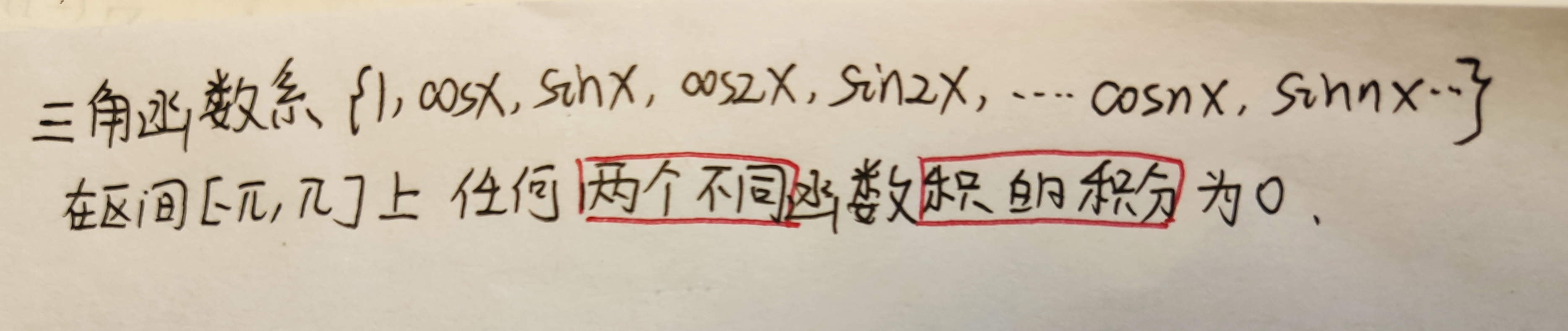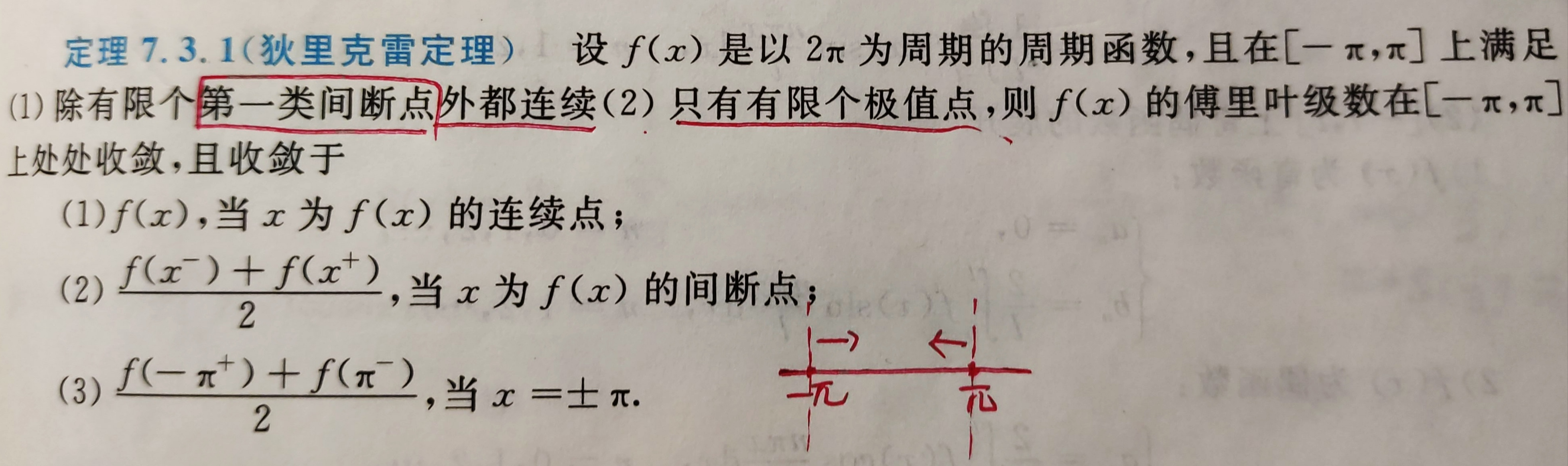在关于原点对称的周期上，对延拖以后的那个函数用收敛定理。
算系数时，用不到延拖的。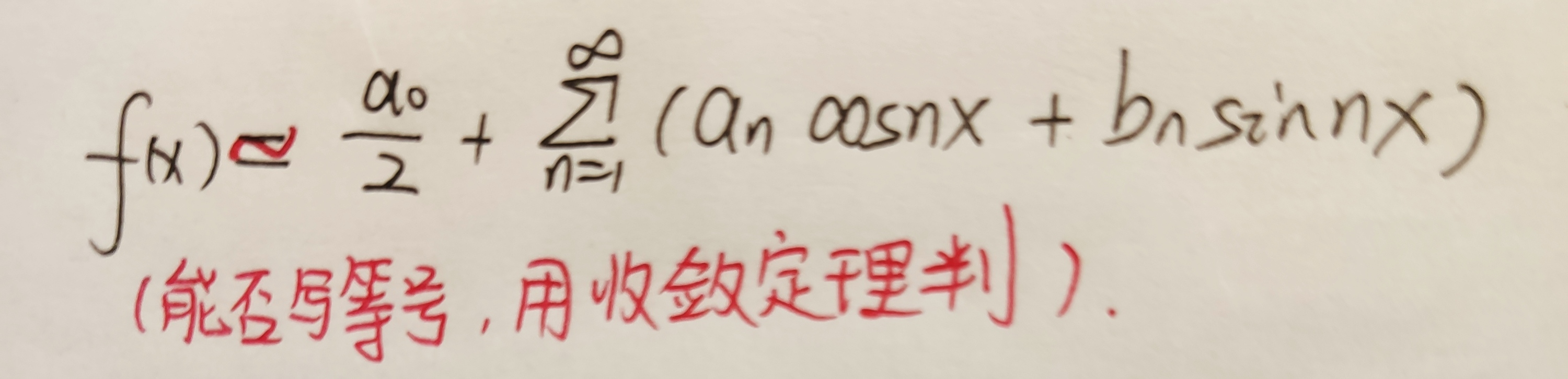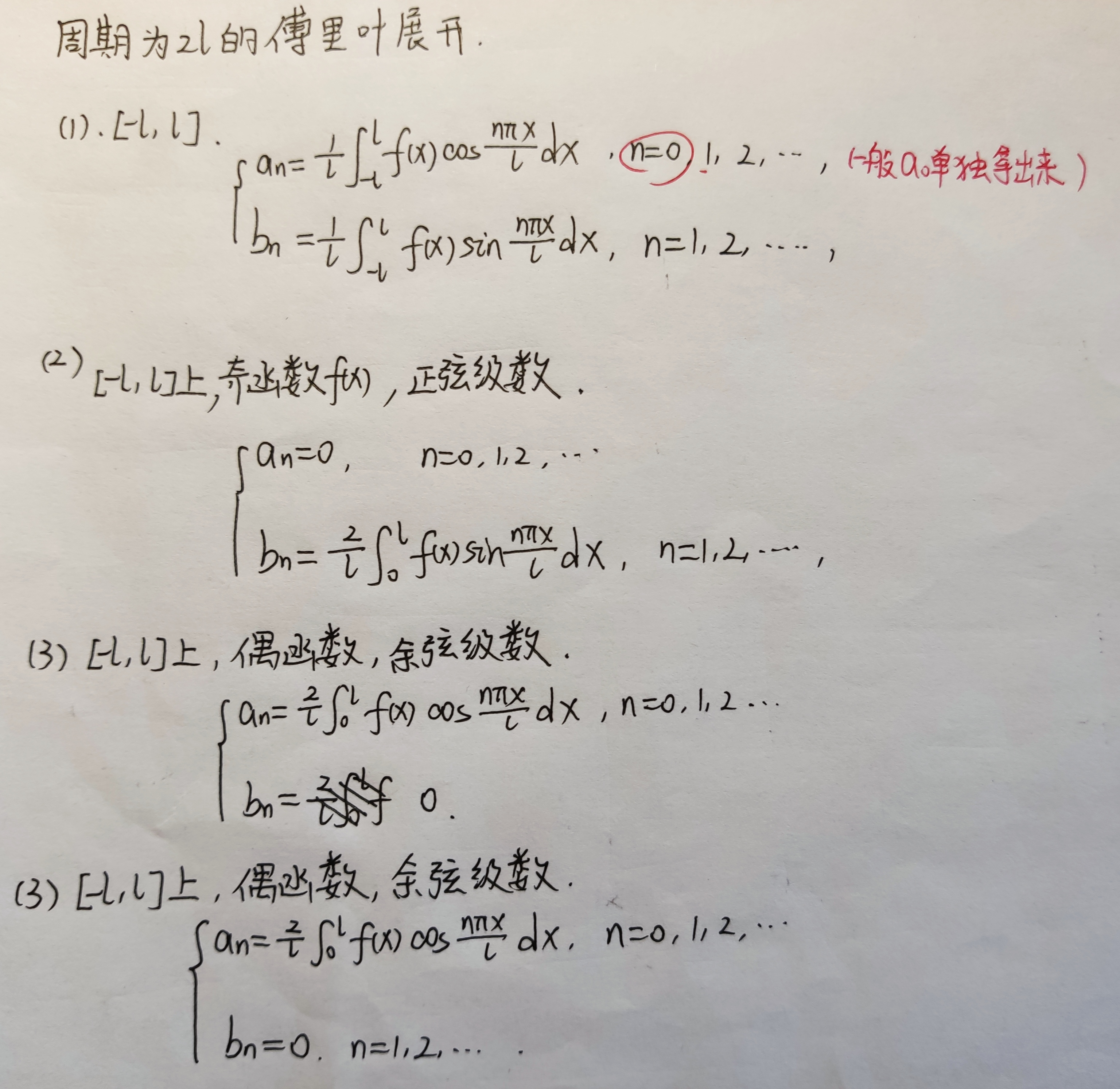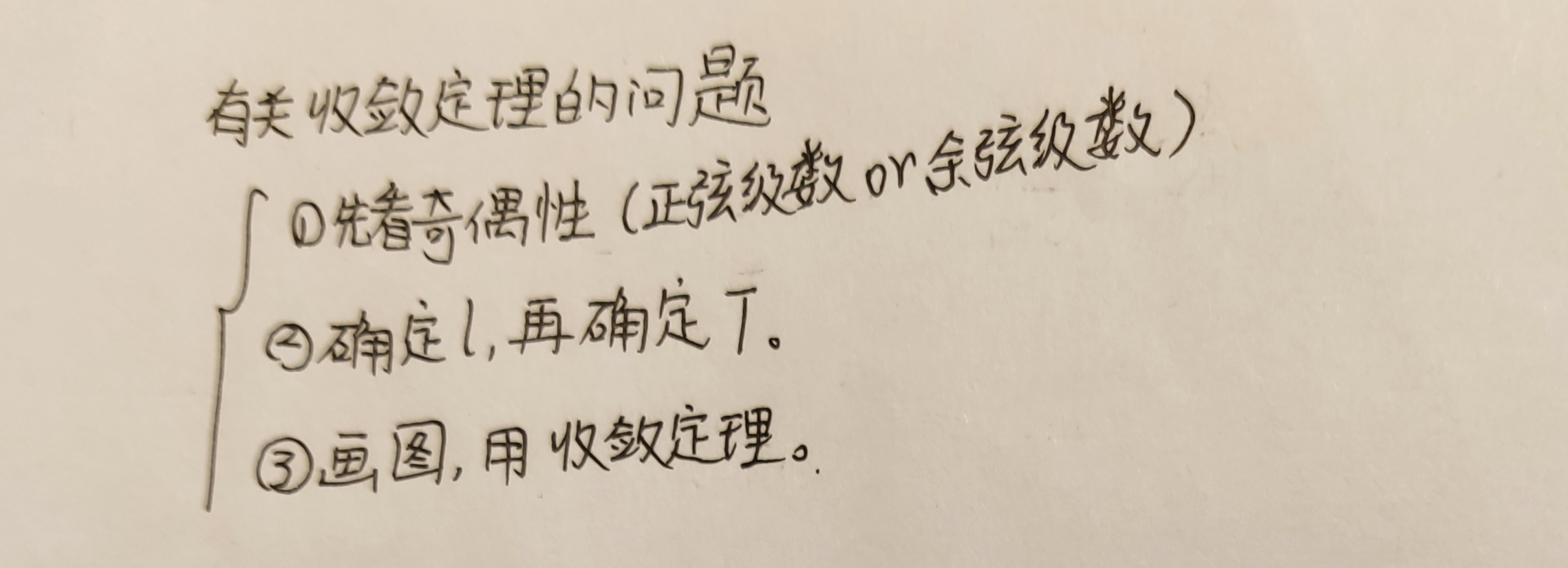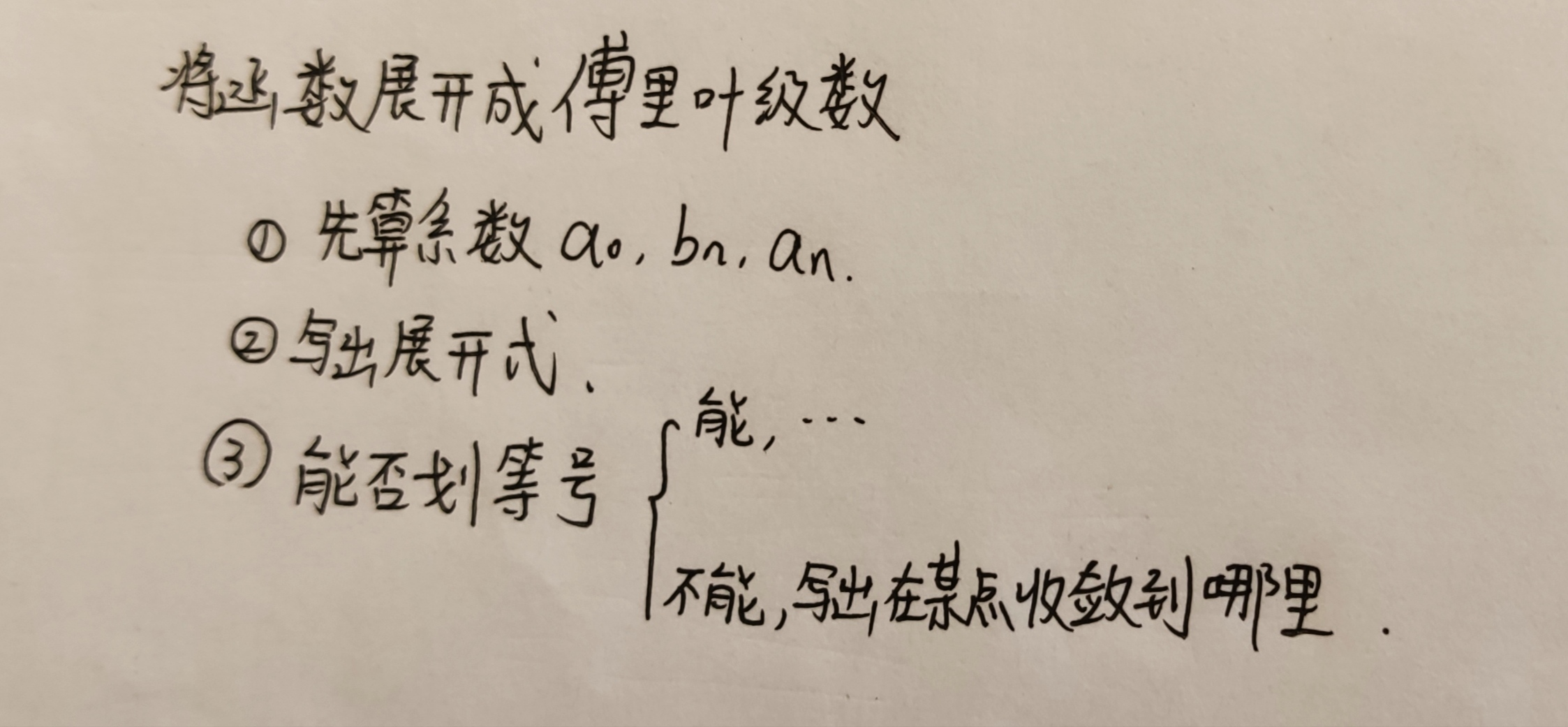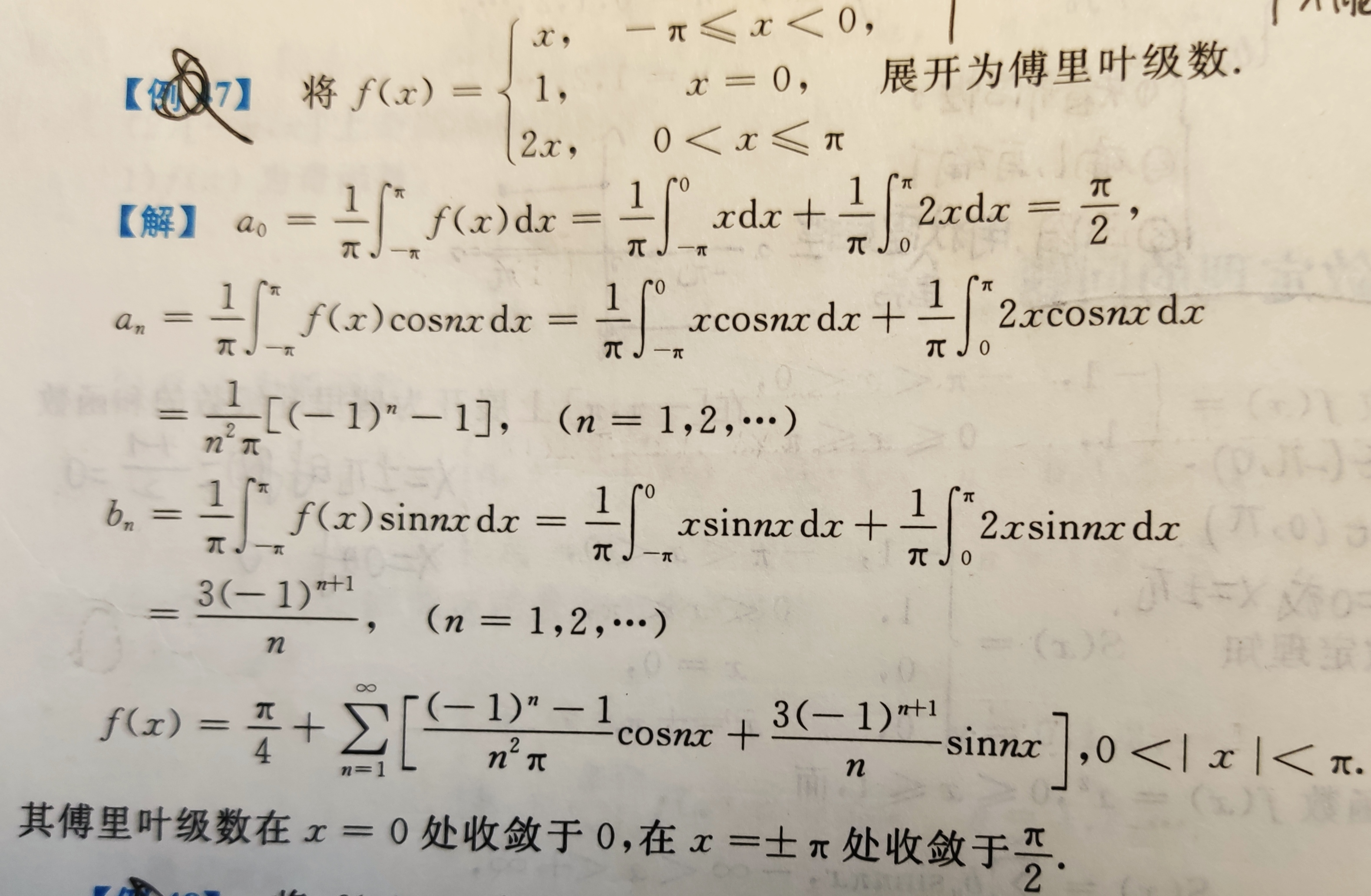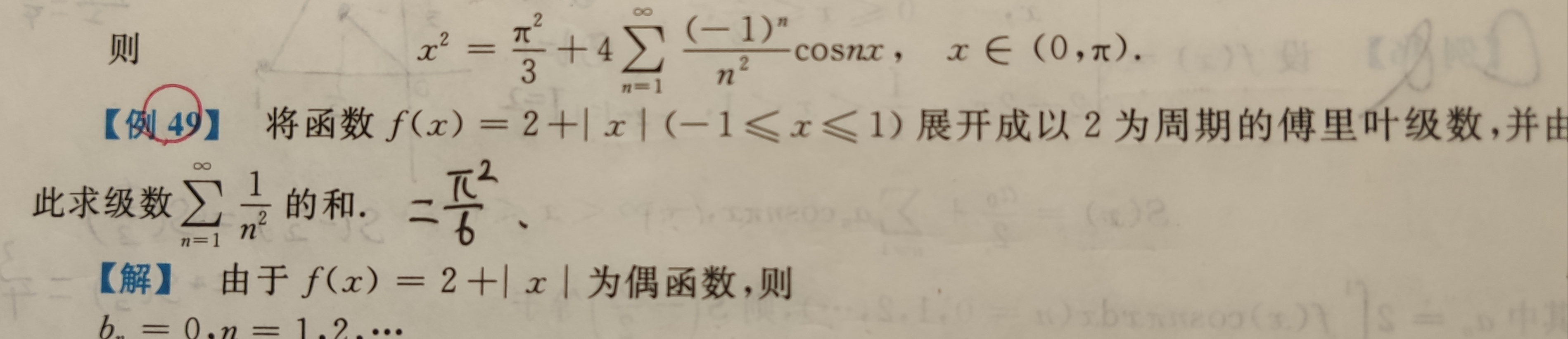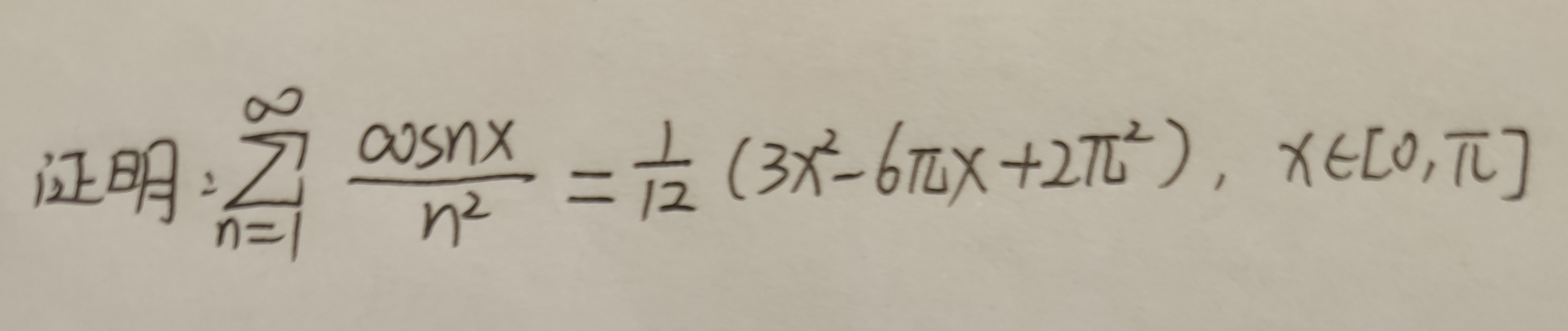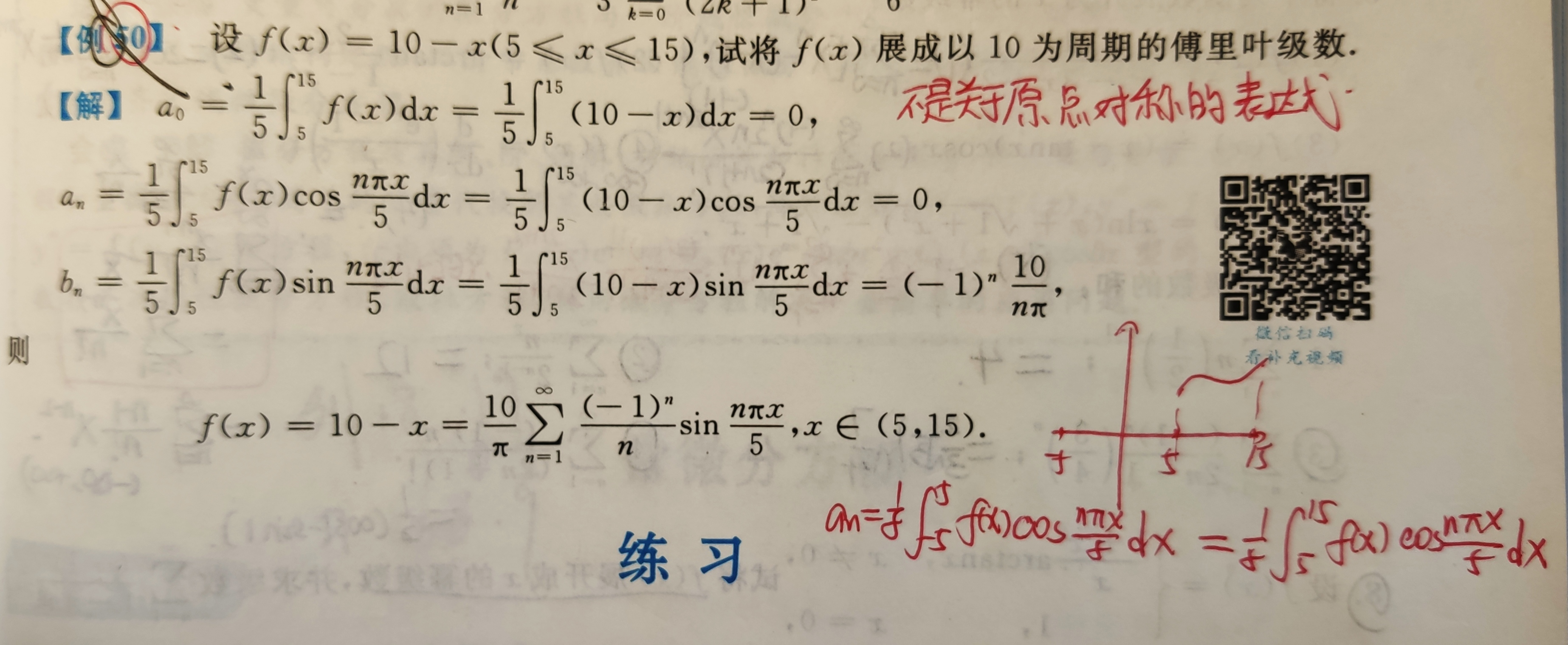展开全文• 常数项级数级数的分类级数收敛数项级数函数项级数常数项级数性质两个重要级数正向级数正项级数审敛法交错级数莱布尼茨审敛法绝对收敛与相对收敛常数项级数审敛法 级数的分类 一、 1、常数项级数 2、幂级数 3、傅里叶...
常数项级数级数的分类级数收敛数项级数函数项级数常数项级数性质两个重要级数正向级数正项级数审敛法交错级数莱布尼茨审敛法绝对收敛与条件收敛常数项级数审敛法
级数的分类
一、
1、常数项级数
2、幂级数
3、傅里叶级数

二、
数项级数
函数项级数

级数收敛
数项级数
给定一个数列 ，对它的各项依次用“+”号连接起来的表达式 “a1+a2+…+an ” 称为数项级数，或称为无穷级数，也可以简称为级数，记为 ∑an
数项级数收敛的充要条件：
部分和数列的极限收敛于S

函数项级数
设有一函数序列
u1(x) , u2(x)  …  un(x)，
若 ui(x)  (i=1,2…n) 在I上有定义
则称∑ui(x)=u1(x) + u2(x)  +  …  +  un(x)   (i=1,2…∞)为函数项级数
对于任意 x0∈I，有∑ui(x0) 可表示为一个常数项函数
若取x=x0时，∑ui(x0)收敛，则称x0为函数项级数的一个收敛点，若发散，则称为发散点
所有发散点的集合称为该级数的发散域，收敛的集合称为收敛域
常数项级数
什么叫做常数项？
不含字母的就叫做常数项
定义：给定一个数列 a1,a2,a3…an
s=a1+a2+…+an (n->∞)称为常数项级数
Note：常数项级数是数项级数
性质
1、收敛的级数 每一项乘以常数k，依然收敛
收敛级数 Sn=a1+...+an
则 kSn=k·a1+...+k·an 收敛

2、收敛级数增加、减少、改变有限项，级数仍然收敛
Note：虽然不改变敛散性，但是可能会改变数值

3、级数Sn=a1+...+an 收敛，Kn=b1+...+bn收敛
则 Sn+Kn=a1+b1+...+an+bn;依然收敛
Note：收敛+发散—发散；发散加发散—不一定发散
例如：(-1)^n -(-1)^n

4、级数收敛的必要条件 an=0（n->∞）
Note：若an≠0（n->∞）级数一定发散
若an=0（n->∞） 级数不一定收敛

5、一个级数收敛，添加任意多括号后一定收敛；
一个级数添加任意多括号后收敛，原级数不一定收敛（括号提高收敛性）

两个重要级数
一、p级数
形如：1/(n^p)
当p≤1时，级数发散
当p＞1，级数收敛
特别的，当p=1时，称为调和级数

二、几何级数
形如：an=a·(q^n) (a≠0)
当q≥1时，发散
当q＜1，收敛，收敛于  “首项/(1-公比)”

正向级数
任意 an >=0
正向级数收敛的充要条件：
lim an=0 （n->∞)  且 部分和数列有界 即：lim Sn= S (n->∞)
等价于 ∑a2n收敛 且 ∑a(2n-1)收敛  (n-<∞)

正项级数审敛法
一、比较法
基本形式:
Sn=a1+..+an;收敛
若Kn=b1+..+bn;
且an>=bn 则Kn收敛

反之：
若Kn 发散
且an≥bn 则Sn发散

极限形式：
Sn=∑an 收敛
Kn=∑bn
若 lim an/bn=l (0<l<∞) (n->∞);则Kn与Sn敛散性相同

推广
若 lim an/bn=0  (n->∞) bn收敛 则an收敛
若 lim an/bn=∞  (n->∞) bn发散 则an发散

二、比值法
设 lim a(n+1)/an = ρ (n->∞)
若 ρ > 1 则发散；
若 ρ < 1 则收敛；
Note: ρ=1 无法判断

三、根值法
设 lim √an= ρ (n->∞)
若 ρ > 1 则发散；
若 ρ < 1 则收敛；
Note: ρ=1 无法判断

四、积分审敛法
若{an}递减，则令f(n)=an;
∫f(x)dx (1->∞) 与an {an}敛散性相同

Noet:
1、先判断是否满足级数收敛的必要条件，若不满足则发散
2、若级数一般项可以表示为数列相邻两项之差，使用定义判断敛散性
3、给出一个不够具体的正项级数，则使用级数的性质以及正向级数审敛法判断
4、给出一个具体的正项级数，使用审敛法来判断，有阶乘的使用比值审敛法，
有n次方的用根植审敛法，有对数的使用积分审敛法，其余使用比较审敛法

交错级数
形如Sn=∑(-1)^n· an  （an>0)
莱布尼茨审敛法
若:
①an>0
②{an} 递减
③lim an = 0 (n->∞)

则 Sn 收敛

Note:
②  是充分条件，而非必要
绝对收敛与条件收敛
Sn=a1+…+an 收敛
若有S’ n = |a1|+…+|an| 收敛 则称 Sn绝对收敛
否则 称Sn 条件收敛
Note： 加绝对值号会提高发散性；
若Sn是正向级数且收敛，其绝对收敛
绝对收敛与条件收敛的关系：
若{an}绝对收敛，则 ∑an 收敛 反之不对；
若 ∑an发散， 则 ∑|an| 一定发散；

常数项级数审敛法
一、
1、先判断是否满足级数收敛的必要条件，若不满足则发散

二、先判断是否为正项级数，若是
1、若级数一般项可以表示为数列相邻两项之差，使用定义判断敛散性
2、给出一个不够具体的正项级数，则用级数的性质以及正向级数审敛法判断
3、给出一个具体的正项级数，使用审敛法来判断，有阶乘的使用比值审敛法，
有n次方的用根植审敛法，有对数的使用积分审敛法，其余使用比较审敛法

三、若不是正向级数，判断是否为交错级数，若是，使用莱布尼茨审敛法

四、其他的常数项级数用绝对收敛和条件收敛判断敛散性

Note：
若 ∑an 收敛 ，则∑|an|、∑(an)^2 、∑an·a(n+1)、∑(-1)^n·an   、∑an·[(-1)^n]/n ·不一定收敛


展开全文• 一、傅里叶级数 1.1 对周期函数进行分解的猜想 拉格朗日等数学家发现某些周期函数可以由三角函数的和来表示，比如下图： 而另外一位数学家猜测任意周期函数都可以写成三角函数之和。 1.2 分解的思路 1.2.1 常数项 ...
一、傅里叶级数
1.1 对周期函数进行分解的猜想
拉格朗日等数学家发现某些周期函数可以由三角函数的和来表示，比如下图：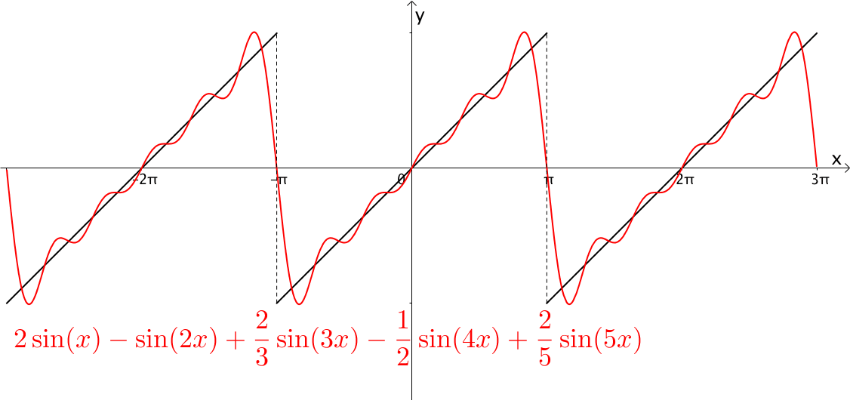而另外一位数学家猜测任意周期函数都可以写成三角函数之和。
1.2 分解的思路
1.2.1 常数项
根据周期函数的定义，常数函数是周期函数，其周期是任意实数，所以，对于$y=C,C\in R$这样的常数函数，分解里面要添加一个常数项与之对应。
1.2.2 其他部分通过$sin(x),cos(x)$进行分解
思路：

$sin(x),cos(x)$是周期函数，进行合理的加减组合，结果可以是周期函数，且它们的微分和积分都很简单。
任意函数都可以分解成奇偶函数之和：
$f(x)={f(x)+f(-x)\over 2}+{f(x)-f(-x)\over 2}=f_{even}+f_{odd}$
$sin(x)$是奇函数，奇函数与奇函数加减永远是奇函数
$cos(x)$是偶函数，偶函数和偶函数加减永远是偶函数

1.2.3 保证组合出来周期为T
之前提到$f(x)$是周期为T的函数，那么怎么保证组合出来的函数周期仍然为T呢？
首先，我们知道$sin(x)$的周期为$2\pi$，$sin(2x)$的周期也是$2\pi$，但是最少周期是$\pi$，很显然，$sin(x),n\in N$的周期都是$2\pi$。
所以更一般的，如果$f(x)$的周期为T，那么$sin({2\pi n\over T}x),cos({2\pi n\over T}x),n\in N$这些函数的周期也为T，再将这些函数进行加减，就保证了得到的函数周期也为T。
1.2.4 调整振幅
假如有如下这个函数，周期为$2\pi$：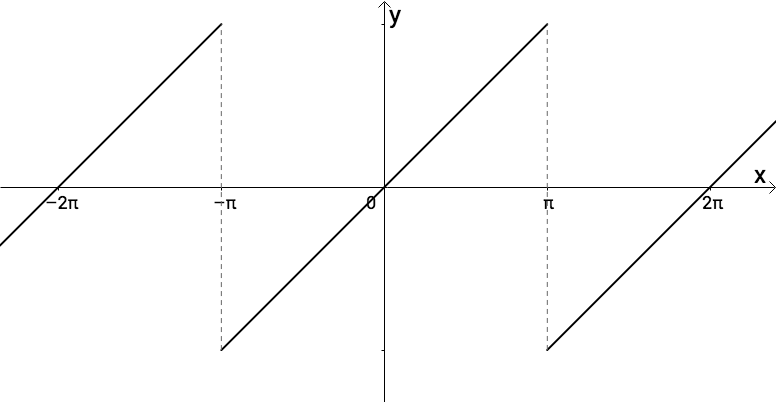现在我们也有一些周期为$2\pi$的函数，比如$sin(x),sin(2x),sin(3x),sin(4x),sin(5x)$：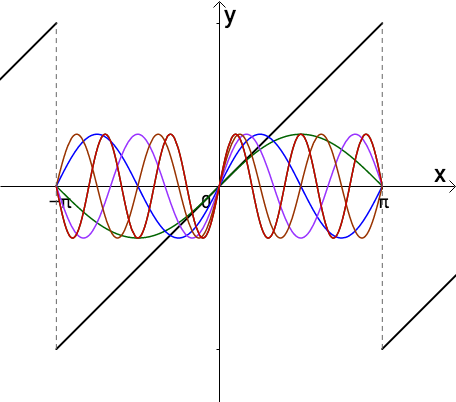通过调整振幅可以让它们慢慢接近目标函数，比如$sin(x)$处处小于目标函数：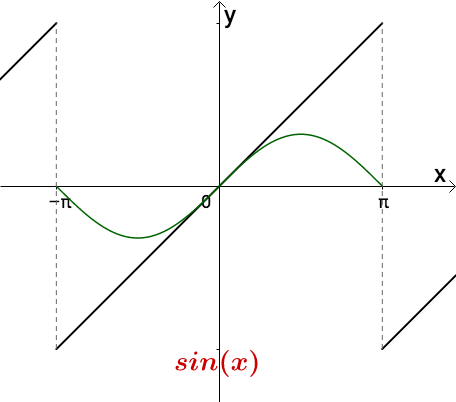将其振幅增大一倍可得：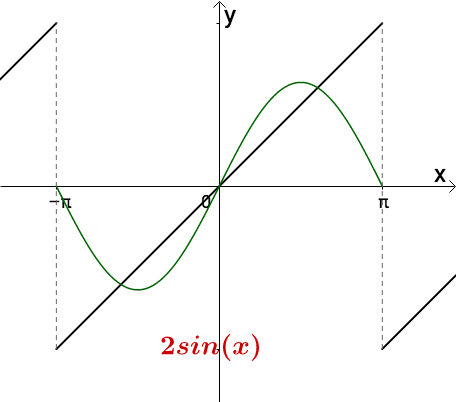此时$2sin(x)$有的地方超过去了，所以从周期为$2\pi$的函数中选择一个，减去一点，可以得到$2sin(x)-sin(2x)$：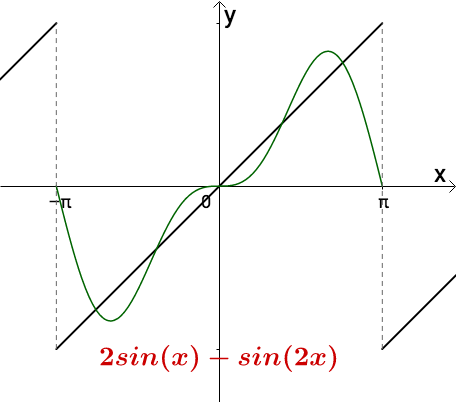通过调整振幅，加加减减，我们可以慢慢接近目标函数：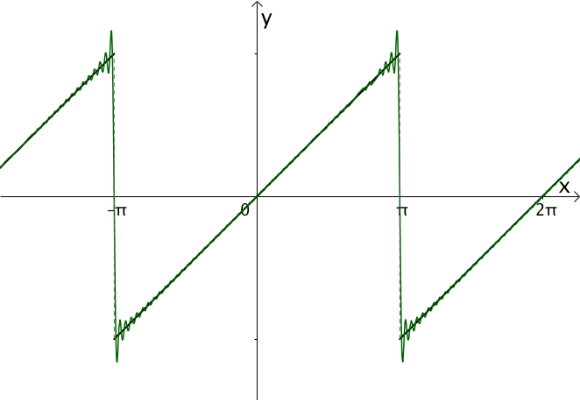1.2.5 综上，构造出来的三角函数和类似于如下的样子：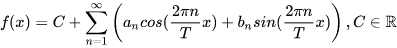这符合之前的分析：

有常数项
用奇函数和偶函数组合出任意函数
周期为T
调整振幅，逼近原函数

1.3 $sin(x)$的另外一种表示方法
1.3.1 $e^{iwt}$
欧拉公式：
$e^{i\theta}$代表复平面一个夹角为$\theta$的向量：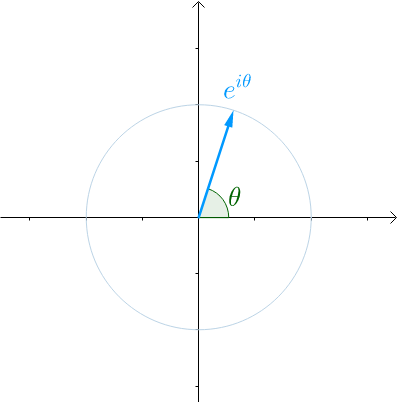当$\theta$不再是常数，而是代表时间的变量t的时候：$e^{i\theta }\to e^{it}$，随着时间t的增长，$\theta$不断增大，这个向量就会旋转起来，$2\pi$的时间会旋转一圈，这就是$T=2\pi$
1.3.2 通过$e^{iwt}$表示$sin(t)$
根据欧拉公式，有：$e^{it}=cos(t)+isin(t)$，所以，在时间轴t上，把e^{it}向量的虚部（也就是纵坐标）记录下来，得到的就是$sin(t)$：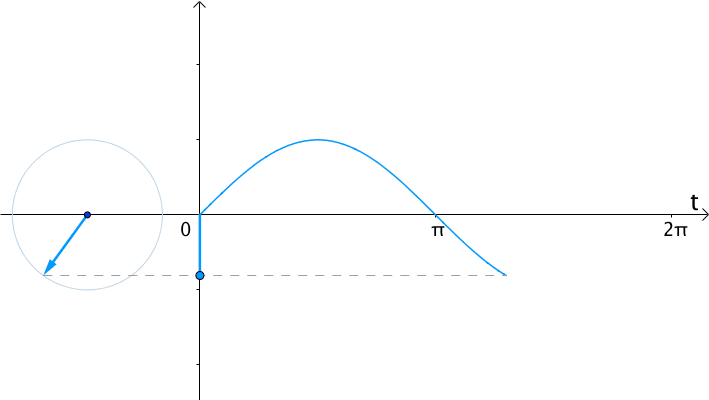如果在时间轴t上，把$e^{it}$的实部（横坐标）记录下来，得到的就是$cos(t)$的曲线：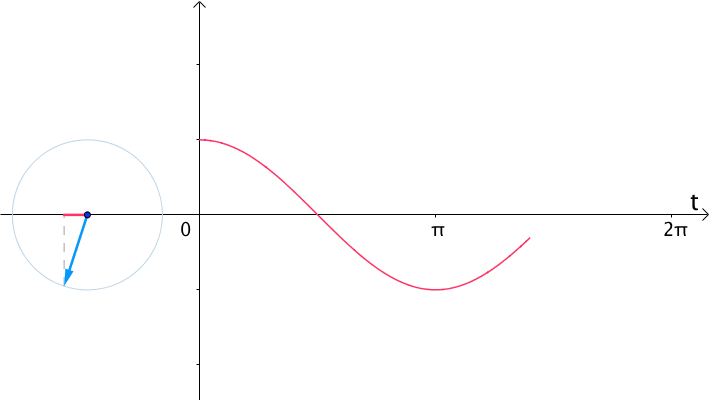更一般的，我们认为有两种看待$sin(x),cos(x)$的角度：
$e^{iwt}\iff\begin{cases}sin(wt)\\cos(wt)\end{cases}$
这两种角度，一个可以观察到旋转的频率，所以称为频域；另一个可以看到流逝的时间，所以称为时域：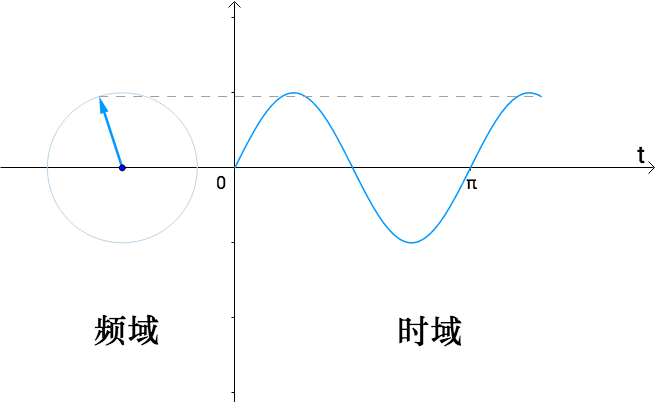1.4 通过频域求系数：
假设有这么一个函数：$g(x)=sin(x)+sin(2x)$是一个$T=2\pi$的函数：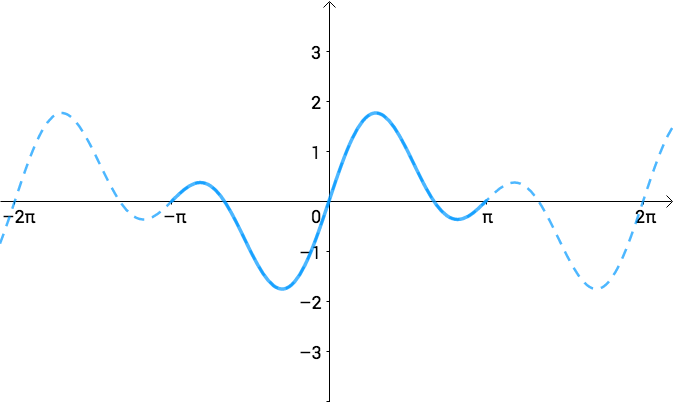如果转到频域去，那么函数$g(x)$就是下面这个复数函数的虚部：$e^{it}+e^{2it}$
首先看下$e^{i\theta}+e^{i2\theta}$，其中$\theta$是常数，很显然这是两个向量之和：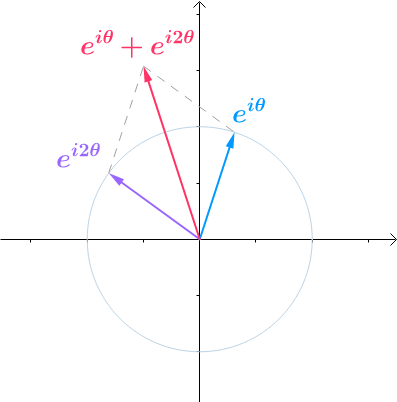将$\theta$换成流逝的时间t，并将虚部记录下来：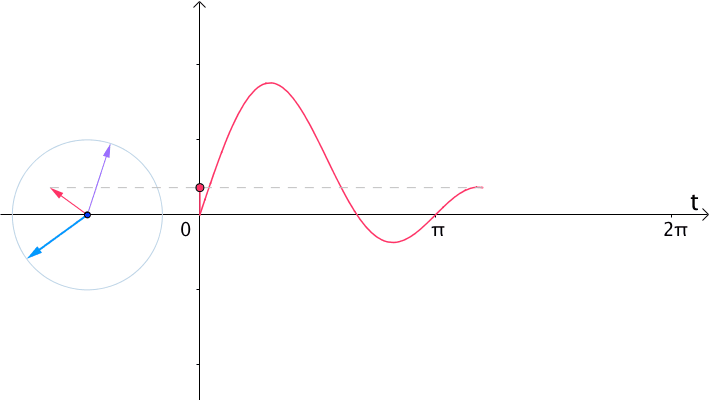令$G(t)=e^{it}+e^{i2t}$，这里用大写的G来表示复数函数，刚才看到$e^{it}$和$e^{i2t}$都是向量，所以上式可以写作：
$\overrightarrow{G(t)}=\overrightarrow{e^{it}}+\overrightarrow{e^{i2t}}$
这里，从线性代数的角度：

$e^{it}$和$e^{i2t}$是基
$G(t)$是基$e^{it},e^{i2t}$的线性组合

$g(t)$是$G(t)$的虚部，所以取虚部的向量分量，很容易得到：
$\overrightarrow{g(t)}=\overrightarrow{sin(t)}+\overrightarrow{sin2t}$，即$g(t)$是基$sin(t),sin(2t)$的线性组合。
那么$sin(t),sin(2t)$的系数，实际上是$g(t)$在基$sin(t),sin(2t)$下的座标了。
1.4.1 如何求正交基的坐标
假设$\overrightarrow{w}=2\overrightarrow{u}+3\overrightarrow{v}$，其中，$\overrightarrow{u}=\begin{pmatrix}1\\1\end{pmatrix},\overrightarrow{v}=\begin{pmatrix}-1\\1\end{pmatrix}$
通过点积：$\overrightarrow{u}\centerdot\overrightarrow{v}=0$，可知这两个向量正交，是正交基，通过点积可以算出$\overrightarrow{u}$的系数（对于正交基才可以这么做）：
${\overrightarrow{w}\centerdot\overrightarrow{u}\over\overrightarrow{u}\centerdot\overrightarrow{u}}={(-1,5)\centerdot(1,1)\over(-1,1)\centerdot(-1,1)}=2$
1.4.2 如何求$sin(nt)$基下的坐标
首先抛出一个结论，函数向量的点积是这么定义的：
$\overrightarrow{f(x)}\centerdot\overrightarrow{g(x)}=\int_0^Tf(x)g(x)dx$
其中，$f(x)$是函数向量，$g(x)$是基，T是$f(x)$的周期。
那么对于$g(x)=sin(x)+sin(2x)$，其中$g(x)$是向量，$sin(t),sin(2t)$是基，周期$T=2\pi$。根据刚才内积的定义：
$\overrightarrow{sin(t)}\centerdot\overrightarrow{sin(2t)}=\int_0^{2t}sin(t)sin(2t)dt=0$，所以这是一个正交基，那么根据刚才的分析，可以这么求坐标：1.4.3 更一般的情况
对于之前的假设，其中$f(x)$的周期为T：可以改写成这样：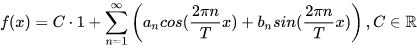也就是说向量$f(x)$的基为：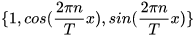这里1也是一个基，那么可以得到：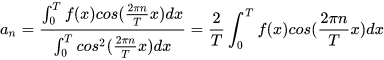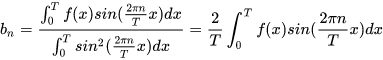C也可以通过点积来表示，最终可以得到：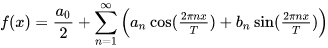其中：二、从傅里叶级数到傅里叶变换
2.1 傅里叶级数
2.1.1 傅里叶级数是向量
从代数上看，傅里叶级数就是通过三角函数和常数项来叠加逼近周期为T的函数$f(x)$：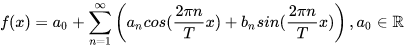这一过过程，实际上是把$f(x)$当作了如下基的向量：那么上面的式子就可以解读为：以一个例子来说明，比如一个$T=2\pi$的方波$f(x)$，可以粗略的写作$f(x)\approx1+{4\over\pi}sin(x)$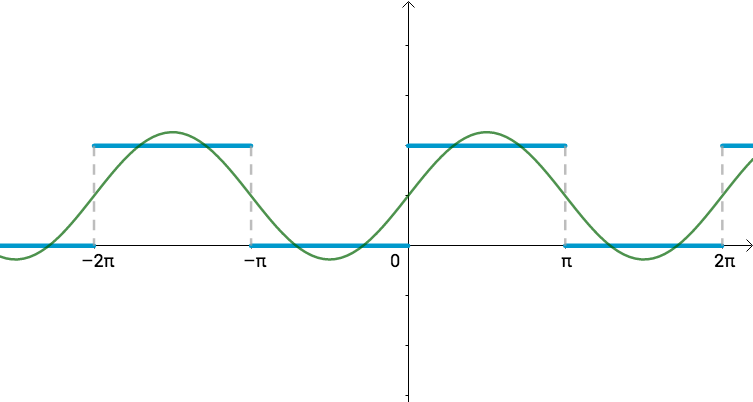我们可以认为$f(x)\approx1+{4\over\pi}sin(x)$这个函数的基为$\{1,sin(x)\}$，则$f(x)$相当于向量$(1,{4\over\pi})$，画到图上如下，注意横纵坐标：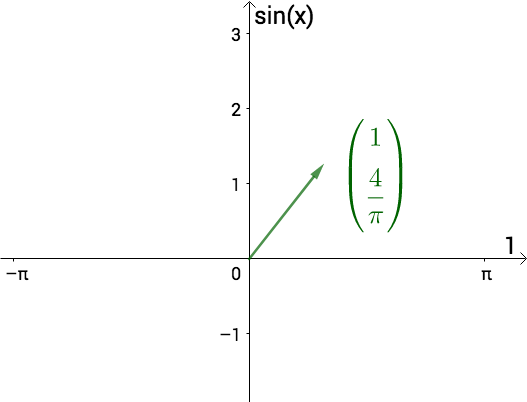2.1.2 频域图
再增加几个三角函数：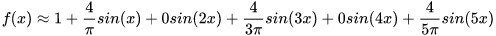此时从几何上来看，图像更为接近：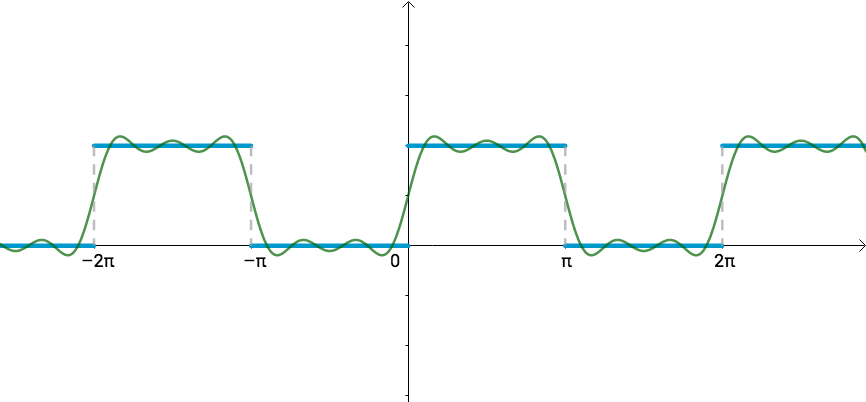这时的基为：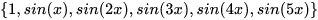对应的向量为：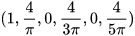六维的向量我们是没有办法通过坐标图来表示的，因此数学家使用了一个频域图来表示这个向量：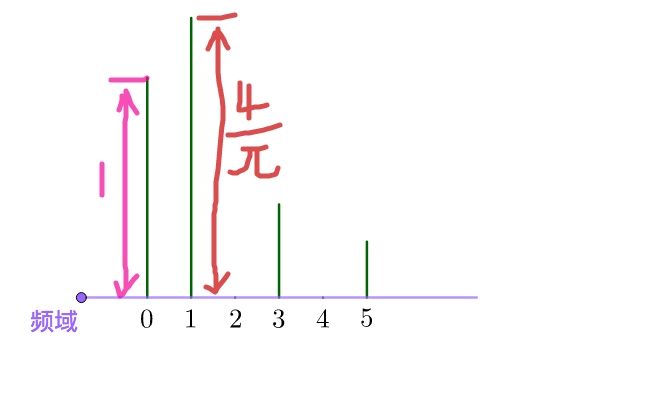上图中的0，1，2，3，4，5分别代表了不同频率的正弦波函数，也就是之间的基：
$0Hz\iff sin(0x)\quad3Hz\iff sin(3x)...$
而高度则代表在这个频率上的振幅，也就是这个基上的坐标分量。
这里举的例子只有正弦函数，余弦函数其实也需要这样一个频谱图，也就是需要两个频谱图，此外还有一种结合正弦和余弦的方式，这个放在后面。
原来的曲线图就称为时域图，往往把时域图和频域图画在一起，这样才能较为完整的反映傅里叶级数。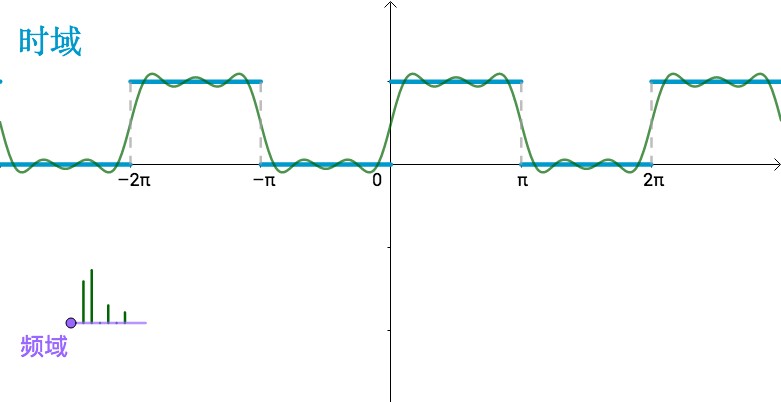不管是时域还是频域，其实反映的都是同一个直线，只不过一个用了函数的观点，而另一个用了向量的观点。
2.2 非周期函数：
以上关于傅里叶级数的说明都是基于周期函数，假如有如下一个非周期函数，那么傅里叶级数该怎么处理？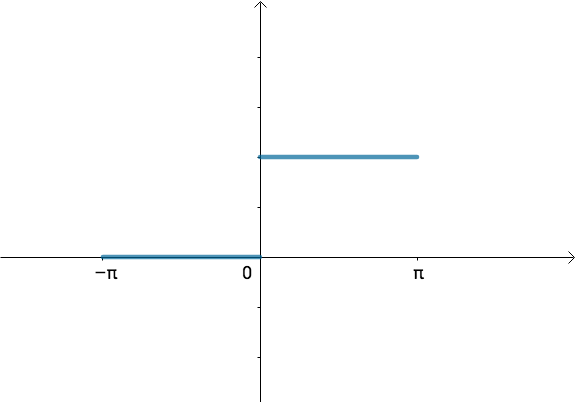我们可以变换一下思路，如果刚才方波的周期：
$T=2\pi\to T=\infin$
那么可以得到一个如下的函数：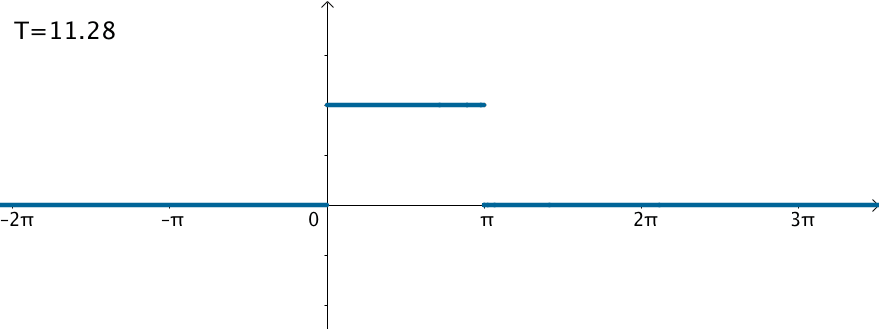在这样的思路下，就可以使用三角级数来逼近这个函数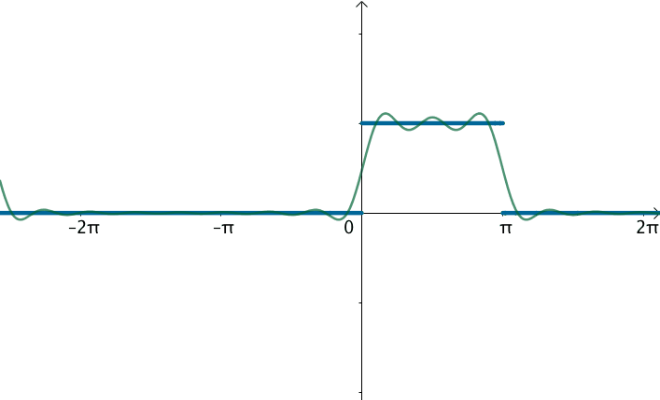观察下频域，之前说过，对于周期为T的函数$f(x)$,其基为：刚才举的方波$T=2\pi$，对应的基就为（没有余弦波）：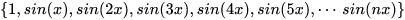对应的频率就是：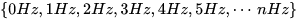按照刚才的思路，如果T不断变大，比如让$T=4\pi$，对应的基就为（没有余弦波）：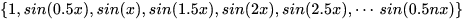对应的频率就为：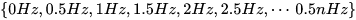和刚才相比，频率更加密集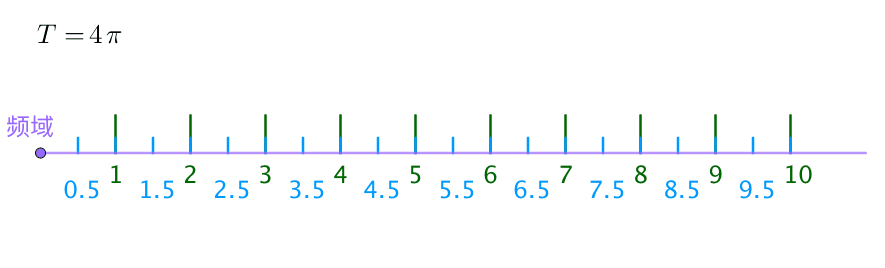之前方波的频域图，画了前五十个频率，可以看到随着$T$不断变大，这50个频率越来越集中：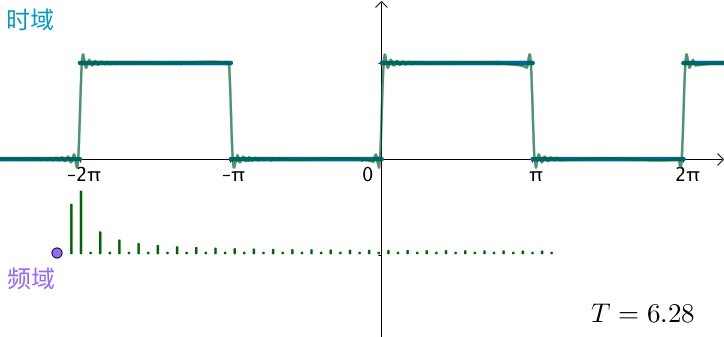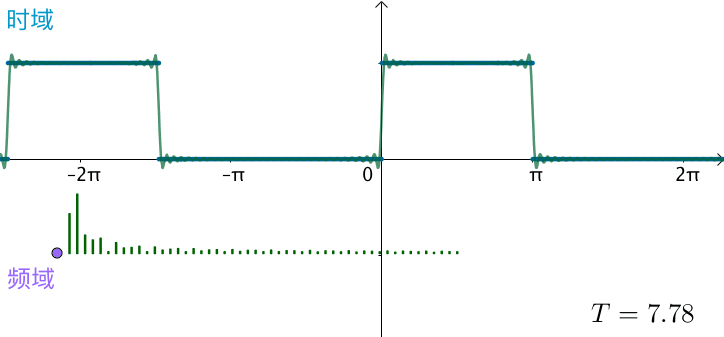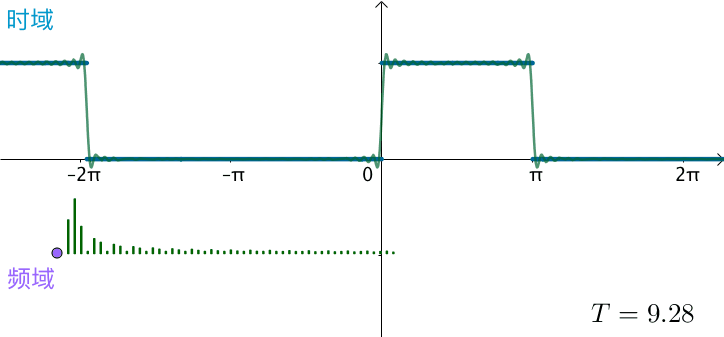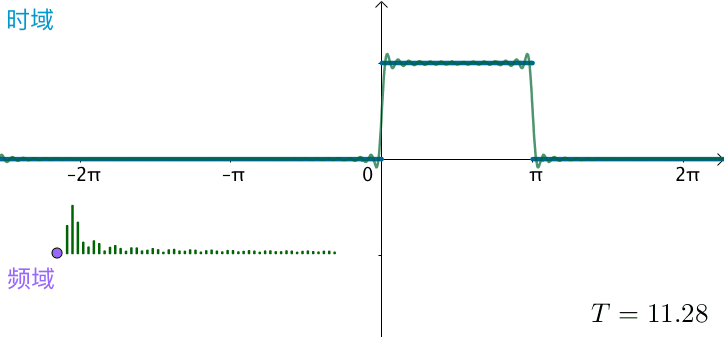可以想象，如果真的：$T=2\pi\to T=\infty$，这些频率就会变得稠密，直至连续，变为一条频域曲线：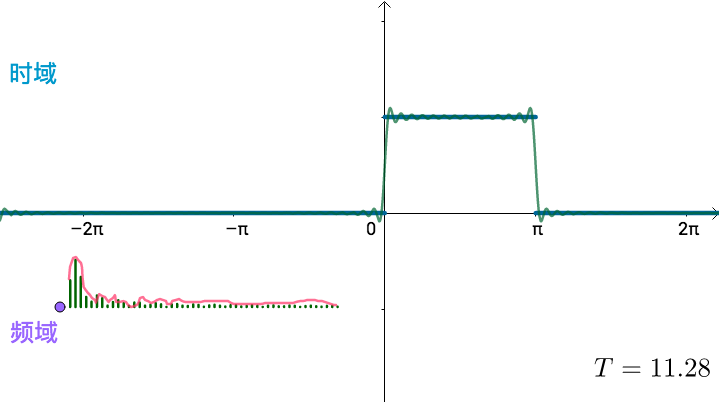傅里叶变换就是，让$T=\infty$，求处上面这根频域曲线
2.3 傅里叶变换
傅里叶级数是：这里有正弦波和余弦波，画频域图不方便，通过欧拉公式，可以转变为复数形式：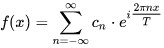其中：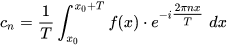复数形式也是向量，可以理解为：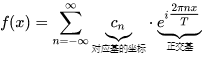周期推向无穷的时候可以得到：其中$F(w)$：$F(w)$就是傅里叶变换，得到的就是频域曲线
下面两者称为傅里叶变换对，可以相互转换：$f(x)\iff F(w)$，正如之前所说的，这是看待同一个数学对象的两种形式，一个是函数，一个是向量。


展开全文• 其中，C是常数项，也可理解为振幅为0的三角函数，其频率和相位任意；，和表示正弦函数k的振幅、角频率和相位（为基频，即为角频率的最小单元）。k取值从1到无穷大。因此，傅里叶级数也就是一个无穷级数。 接着我们...
数学家傅里叶猜想任何一个周期函数均可分解为一系列不同振幅、不同频率和不同相位的正弦函数的组合（由此，复杂的周期函数可分解为若干简单的三角函数，易于分析和处理）。即：

其中，C是常数项，也可理解为振幅为0的三角函数，其频率和相位任意；，和表示正弦函数k的振幅、角频率和相位（为基频，即为角频率的最小单元）。k取值从1到无穷大。因此，傅里叶级数也就是一个无穷级数。

接着我们利用两角和公式sin(A+B)=sin(A)cos(B)+cos(A)sin(B)对上式做分解，得到：

令和，得到f(t)的傅里叶级数表达式：

如果上式中的系数C、ak和bk可解，则f(t)可分解为一系列简单的三角函数。

我们选择正弦函数k中最大的周期作为积分区间（保证所有的三角函数在此区间内积分为0）。对f(t)的傅里叶级数表达式在内积分得：

因此，系数C得解：

接着f(t)的傅里叶级数表达式左右两边同时乘以，得到：

对上式在内积分得：

因此，系数得解：

接着f(t)的傅里叶级数表达式左右两边同时乘以，得到：

对上式在内积分得：

因此，系数an得解：

至此，已经得到傅里叶级数中各系数的表达式（如下），只要它们可积分，即C、an和bn可解，那么就可得到了函数f(t)的傅里叶级数。

傅里叶级数的复数形式

为此，我们需要引入欧拉公式。其构建了三角函数和复数之间的桥梁，如下：

其中，。替换上式中的x为-x，得到。得到：

将上式代入三角函数形式的傅里叶级数中：

观察上式，可以得到傅里叶级数的复数形式：

时：

k=0时：

时，令j=-k：

至此，已经得到复数形式的傅里叶级数中系数的表达式（如下），只要它们可积分，即可解，那么就得到了函数f(t)的复数形式的傅里叶级数。

其中，

https://blog.csdn.net/u012841922/article/details/81335270
展开全文• 三角函数形式的傅里叶级数：在直流分量（常数项）上叠加不同频率的正弦波来近似周期函数。 一、基本形式： 函数的周期为，角频率为，频率，傅里叶级数展开表达式为： ....(1) 其中，n为正整数，各次谐波成分...
• 傅里叶级数的公式： f(x)是一个周期为T的函数； 1.常数项C : g(x) = C存在两种特性： 1）周期函数，并且周期任意； 2）调节函数值； 2.sinx cosx: 1）存在周期性； 2）...
• 1. 总览分类将级数的内容按上...三角级数部分，主要是函数展开成三角级数（即傅里叶级数）。2. 常数项级数概念：给定一个数列那么由这数列构成的表达式叫做常数项无穷级数，简称常数项级数，记为。一般项，部分和，...
• 常数项级数 函数项级数：函数列 收敛点，发散点 收敛域，发散域 一致收敛性 傅里叶级数 时域 -》 幅度-频率 -》 初始相位-频率 正弦函数的叠加 所有周期函数都可以由三角函数叠加而得 三角函数系的正交性 1, sin x, ...
• 1，常数项级数 将有无数个元素的数列写成所有元素相加的形式，就叫做常数项无穷级数，简称常数项级数。 无穷级数的发散和收敛性质可以参考数列发散和收敛的性质。 2，幂级数 将数列中的常数换成函数，就组成了函数项...
• 单看那个①式，就是把周期函数f(t)描述成一个常数系数a0、及1倍ω的sin和cos函数、2倍ω的sin和cos函数等、到n倍ω的sin和cos函数等一系列式子的和，且每都有不同的系数，即An和Bn，至于这些系数，需要用积分来解...
• 第一节 常数项级数的概念和性质第二节 常数项级数的审敛法第三节 幂级数第四节 函数展开成幂级数第五节 函数的幂级数展开式的应用第六节 函数项级数的一致收敛性及一致收敛级数的基本性质第七节 傅里叶级数第八节 ...
• 无穷级数总结：无穷级数包括常数项级数和函数项级数，我们主要讨论常数项级数的性质及其收敛判别法，函数项级数里面的幂级数的收敛半径与收敛域，幂级数的和函数，幂级数的展开及傅里叶级数。其实，无论哪种级数，...
• 无穷级数 常数项级数 函数项级数 幂级数 傅里叶级数无穷级数 函数项级数
• 第一节 常数项级数 10.1 级数的概念与性质 10.2 级数的审敛准则 10.3 常考题型与典型例题 第二节 幂级数 第三章 傅里叶级数
• 级数这部分难点在于和函数，然后傅里叶级数展开那里计算量比较大...第一节 常数项级数温馨提示据说，李永乐复习全书每道题都滚瓜烂熟，可以有120的潜力。那么，我们一定要加油掌握每一道题呀。学习目标：掌握知识点...
• 级数的考查形式：选择、填空、解答题考查要点：常数项级数的敛散性判别、幂级数的收敛半径和收敛域、幂级数的展开和求和、傅里叶级数（只数一要求）。其中常数项级数的敛散性判别主要以选择题形式考查，数一也...
• （还是可以由常数项叠加正弦波组成，只不过叠加的正弦波间隔频率变小。） 周期趋于无限大，则谱线的间隔趋于无限小，这样，离散频谱就变成连续频谱了。同时，由于周期T趋于无限大，谱线的长度趋于零。也就是说，由...
• 　习题12-1　常数项级数的概念和性质 　习题12-2　常数项级数的审敛法 　习题12-3　幂级数 　习题12-4　函数展开成幂级数 　习题12-5　函数的幂级数展开式的应用 　习题12-6　函数项级数的一致收敛性及一致收敛级数...
• 一元函数、微积分 微分学 函数、极限、连续 导数与微分 微分中值定理导数的应用 ...常数项级数 幂级数 傅里叶级数 微分方程 一阶方程 可降阶方程 高阶线性方程 参考书：《复习全书基础篇》 ...
• 　《数学·统计学系列：三角级数论》以现代的观点简明而完整地讲述傅里叶级数的基础理论，全书共分7章。第1章讲述预备性知识；第2，3章讲傅里叶级数的性质；第4章讲傅里叶级数的收敛性及其判别法；第5章、第6章讲...
• 吉谱斯现象Gibbs（又叫吉谱斯效应）： 用有限项傅里叶级数表示有间断点的信号时，在间断点附近不可避免的会出现振荡和超量。超量的幅度不会随所取数的增加而减小。只是随着数的增多，振荡频率变高，并向间断点处...
• 又称吉谱斯现象Gibbs 用有限项傅里叶级数表示有间断点的信号时，在间断点附近不可避免的会出现振荡和超量。超量的幅度不会随所取数的增加而减小。只是随着数的增多，振荡频率变高，并向间断点处压缩，从而使它所...
• 12.1.常数项级数 12.1.1.概念与性质 概念：部分和序列的极限 性质 数乘运算 逐项相加、逐项相减 去掉、加上或改变有限项，不改变级数的收敛性 级数任意加括号和不变 级数收敛，则一般项趋于零 12.1.2.审敛法 正项...
• 吉布斯现象Gibbs phenomenon（又叫吉布斯效应）：将具有不连续点的周期函数（如矩形脉冲)进行傅立叶级数展开后，选取有限进行合成。当选取的数越多，在所合成的波形中出现的峰起越靠近原信号的不连续点。当选取...图像处理 高通 网格
• 傅里叶逼近《MATLAB程序设计实践》科学计算...功能：用傅里叶级数逼近已知的连续周期函数。调用格式：[A0，A，B]=FZZ(func,T,n).其中，func为已知函数；T为已知函数的周期；N为展开级数的项数；A0为展开后的常数项；...
• 荣璟 -《充分利用问题性质——例析动态规划的"个性化"优化》 许智磊 -《浅谈补集转化思想在统计问题中的应用》 张 宁 -《猜数问题的研究》 张云亮 -《论对算法的选择》 周 源 -《浅析"最小表示法"思想在字符串...